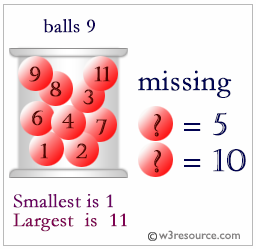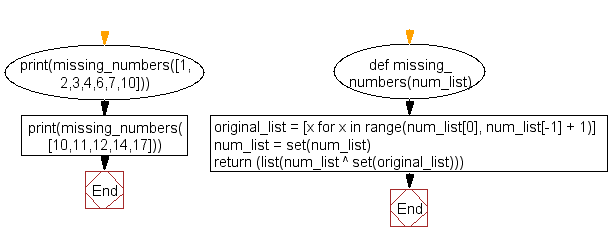﻿ Python: Find missing numbers from a list - w3resource# Python Challenges: Find missing numbers from a list

## Python Challenges - 1: Exercise-8 with Solution

Write a Python program to find missing numbers from a list.

Explanation:Sample Solution:-

Python Code:

``````def missing_numbers(num_list):
original_list = [x for x in range(num_list, num_list[-1] + 1)]
num_list = set(num_list)
return (list(num_list ^ set(original_list)))

print(missing_numbers([1,2,3,4,6,7,10]))
print(missing_numbers([10,11,12,14,17]))
```
```

Sample Output:

```[5, 8, 9]
[13, 15, 16]
```

Flowchart:## Visualize Python code execution:

The following tool visualize what the computer is doing step-by-step as it executes the said program:

Python Code Editor:

What is the difficulty level of this exercise?

Test your Python skills with w3resource's quiz

﻿

## Python: Tips of the Day

Python: Inserting if statements using conditional list comprehensions

```x = [1, 2, 3, 4, 5, 6]
result = []
for idx in range(len(x)):
if x[idx] % 2 == 0:
result.append(x[idx] * 2)
else:
result.append(x[idx])
result
```

Output:

```[1, 4, 3, 8, 5, 12]
```
`[(element * 2 if element % 2 == 0 else element) for element in x]`

Output:

```[1, 4, 3, 8, 5, 12]
```
`[element * 2 for element in x if element % 2 == 0]`

Output:

```[4, 8, 12]
```